# 理解使用递归及堆栈的算法处理八皇后问题

• C语言，这个我想只要工科类的学生，肯定学过，有基本数据结构，三种控制流，输入，输出知识足够。现在有些人，使用C++的环境来写不包含对象的C程序，这会简化C语言在输入输出方面的繁琐学习，值得推荐。而C++的特点是，在C语言的基础上加入面向对象的支持，也就是“类”，说白了就是把原来的结构体里面加入与这些数据结构相关的函数。而把面向对象拆分开，不写成“类”，在某些情况下，可以更好地理解程序的结构。本文在网友分享的八皇后程序基础上，修改为比较好理解的结构化程序。
• 函数。这个主要是理解函数调用的原理，重点是每次调用函数，都会开辟一片新的内存空间，函数参数都会被复制一次。
• 递归。递归的调用可以用函数调用来理解，必须有一个“结束”条件，否则容易造成程序死循环。
• 栈。主要是“后入先出”的思想。如何用数组或者链表来创建栈

#define StackSize 8 /*最多放8个皇后*/
int queen[StackSize][StackSize]={0}; /*棋盘*/

int top=-1;                          /*栈顶指针*/
int data[StackSize];                  /*存储皇后位置*/ 

/*放N皇后的递归函数*/
void Place(int row)//摆放皇后
{
bool Judgement();//位置是否合法
void SeqStack();//关键点1：每一次摆放都会初始化空栈，即栈顶指针=-1
void Output();//输出结果
for (int col=0;col<StackSize;col++)//结束条件为col=8
{
Push(col);//将合法位置入栈
if (Judgement()) //判断位置合法
{
if (row<StackSize-1) //若还没有放到第八个皇后，则递归进行下一个皇后的放置
PlaceQueen(row+1);
else
{             //摆放完8个皇后，输出结果
ans++;  //解数加1
Output(); //打印成功的棋盘
}
}
Pop();//若不符合条件则出栈
}
}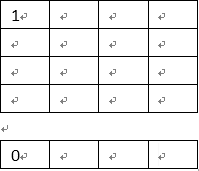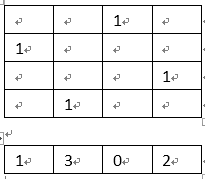data[top]==data[i]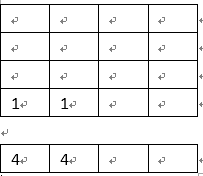abs(data[top]-data[i]))==(top-i)

top-i的值就是皇后之间的非法相对距离，值为0、1、2….，也就是同一条对角线或者斜对角线。data[top]-data[i]为2个皇后的实际的相对距离。比如假设现在place(0)将(0,3)入栈，递归调用place(1)，如果将(1,2)入栈，那就是在右上角的对角线上，必须舍弃，这里data[top]=2，就是刚入栈的(1,2)信息，data=3就是原来栈中所存的皇后位置信息(0,3)，二者之差=1，就是相对距离为1，位置不合法。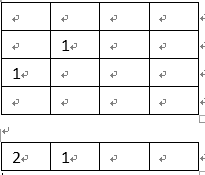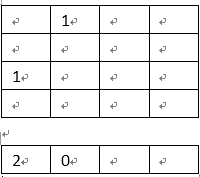#include <iostream>
#include<cmath>
using namespace std;
#define StackSize 8 /*最多放8个皇后*/
int queen[StackSize][StackSize]={0}; /*棋盘*/
int ans=0;                           /*解数*/
int top=-1;                          /*栈顶指针*/
int data[StackSize];                  /*存储皇后位置*/

/*入栈*/
void Push(int x)                                          //入栈操作
{
top++;                                                           //栈顶指针上移
data[top]=x;
}
/*出栈*/
void Pop()                                              //出栈操作
{
top--;                                                           //栈顶指针下移
}

void SeqStack(){top=-1;}                                              //构造函数，初始化空栈

/*放N皇后的递归函数*/
void PlaceQueen(int row)                                //在栈顶放置符合条件的值的操作,即摆放皇后
{
bool Judgement();
void SeqStack();                                    //关键点1：每一次摆放都会初始化空栈
void Output();
for (int col=0;col<StackSize;col++)                              //穷尽0~7，即穷尽列
{
Push(col);
if (Judgement())                                             //判断摆放皇后的位置是否安全
{
if (row<StackSize-1)                                     //若还没有放到第八个皇后，则进行下一个皇后的放置
PlaceQueen(row+1);
else
{
ans++;                                               //解数加1
Output();                                            //打印成功的棋盘
}
}
Pop();                                                       //若不符合条件则出栈
}
}

/*关键点2：判断合法性*/
bool Judgement()
{
for(int i=0;i<top;i++)                                           //依次检查前面各行的皇后位置
if(data[top]==data[i]||(abs(data[top]-data[i]))==(top-i))    //判断是否在同一列同一斜线
return false;                                            /*第一个条件，使每次入栈的数字不同层（行）*/
return true;                                                     /*top-i的值就是皇后之间的非法相对距离，值为0、1、2....，也就是同一条对角线或者斜对角线。data[top]-data[i]为2个皇后的实际的相对距离。*/
}

/*输出棋盘*/
void Output()                                          //将栈的数组形式打印成棋盘形式
{
cout<<"NO."<<ans<<":"<<endl;
for(int i=0;i<StackSize;i++)
{
for(int j=0;j<data[i];j++)
cout<<"- ";                                             //不放置处打印“-”
cout<<"Q";                                                  //放置处打印“Q”
for(int j=StackSize-1;j>data[i];j--)
cout<<" -";
cout<<endl;                                                 //换行
}
cout<<endl;
}
int main()
{
PlaceQueen(0);                                        //从栈底开始赋值
cout<<"the total number of solutions is:"<<ans<<endl;       //输出摆放方法的总数
system("pause");
return 0;
}